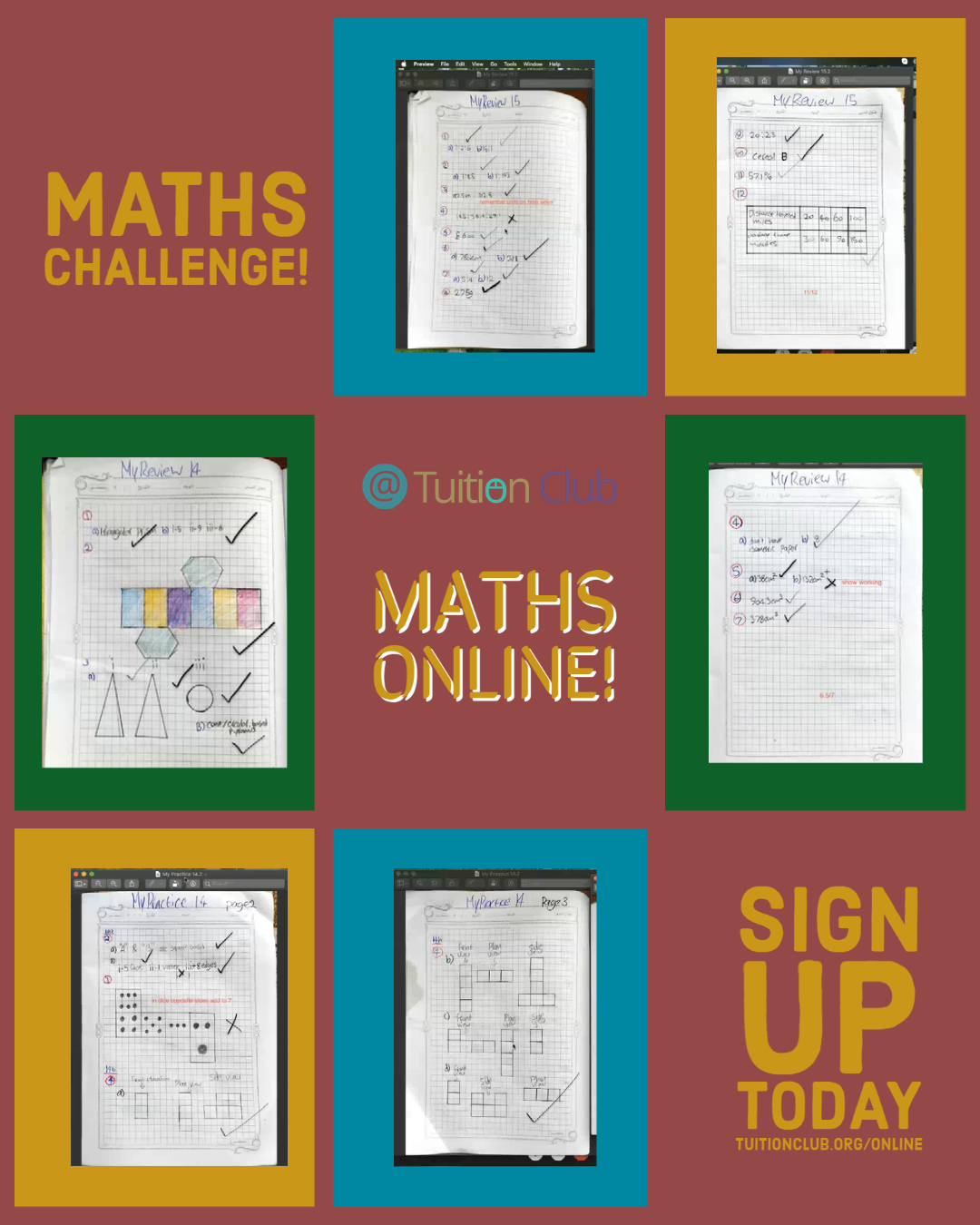# Mathematical Challenges Online!

Our private students have been working through some mathematical challenge questions.

Using the online tools, they have worked through some math problems, questions, and individual assignments on various topics.

Students have been working on Ratio, Proportion, and 3D Shapes.

For each topic, a target was set which was then graded. The students completed exercises on simplifying ratios and worked on solving more complex proportion problems.